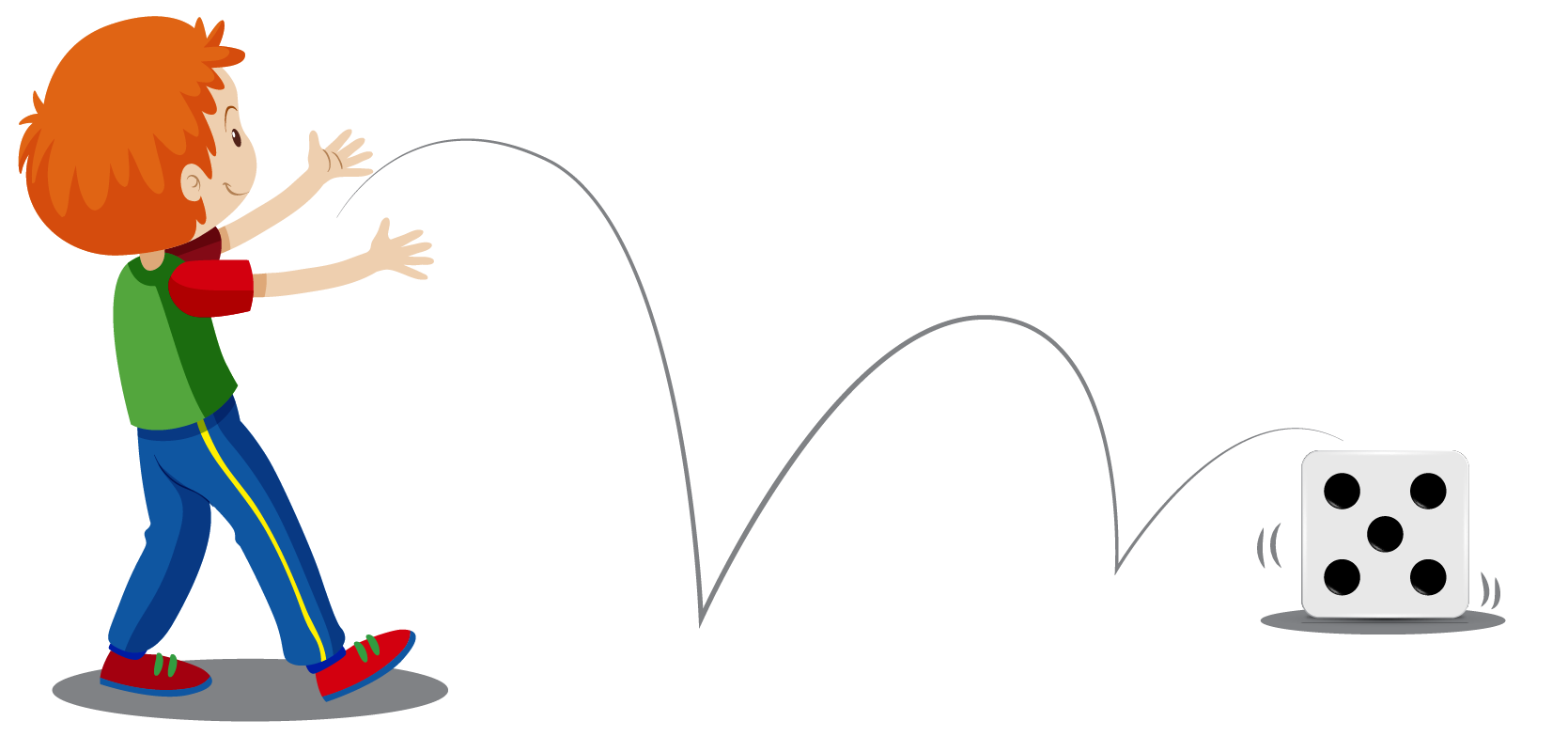# Sample Space

Sample Space

An interesting fact about the theory of probability is that it was created due to a gambler's dispute, and was given by two famous mathematicians, Blaise Pascal and Pierre de Fermat.

This page will tell you more about the concept of sample space in probability, with interesting examples, like the flipping of a coin and the rolling of a dice.

Try this sample space calculator and get started to know more about the concept.

## Lesson Plan

 1 What Is Meant by Sample Space? 2 Tips and Tricks 3 Important Notes on Sample Space 4 Solved Examples on Sample Space 5 Interactive Questions on Sample Space

## What Is Meant by Sample Space?

Sample space, in probability, is referred to as the collection of all possible outcomes in any random experiment. It is denoted by "S".

Since it is a set, it is shown within the braces.

## The Number of Members in a Sample Space

Let's learn how to find the number of members using a few sample space examples.

### Sample space of a coin

Have you ever seen a one cent coin?

You may have noticed that one of its sides, features the 16th U.S. President, Abraham Lincoln.

Let's say Candace flipped two coins. What are the possibilities when she flips the coin?

Each coin could either fall on head or tail right?There isn't any other possibility when a coin is flipped, hence, the sample space of a coin is given as:

Sample space of a coin = {H, T} or {Heads, Tails}.

### Sample space of a dice

Have you ever seen a dice?

It is a cubical toy with 6 faces, showing the numbers in the form of dots.

Let's say, Jeremy rolled a dice. What are the possibilities of the dots that can be seen, when he rolls a dice?

The dice show 1, 2, 3, 4, 5, or 6 dots.Therefore, the sample space of dice is written as {1, 2, 3, 4, 5, 6}Tips and Tricks

• The sample space for a coin tossed 'n' times, or 'n' number of coins, tossed once, is written in the count of $$2^n$$.
• The sample space for a dice rolled 'n' times or 'n' number of dices, rolled once, is written in the count of $$6^n$$.
• When a dice is rolled or a coin is tossed, the outcome considered, is of the side which is seen on the upper face.

## Difference Between Event and Sample Space

Event is a set of outcomes during an experiment, while sample space is all the possibilities that can occur during an experiment.

Hence, the event is a subset of sample space.

Let's look at some sample space examples to understand this better.

### The occurrence of a head when a coin is flipped

The occurrence of a head when a coin is flipped is only once. It can either show heads or tails.

Sample space, S = {occurrence of head, occurrence of tail}

Hence, the case of coin-flipping can be represented as E $$\subset$$ S.

The occurrence of 1 when a dice is rolled

The occurrence of 1 when a dice is rolled is only once. It can show 1, 2, 3, 4, 5 or 6

Event, E= {Occurrence of 1}

Sample space, S = {occurrence of 1, occurrence of 2, occurrence of 3, occurrence of 4, occurrence of 5, occurrence of 6}

Hence, the case of rolling dice can be represented as E $$\subset$$ S.

 Example 1

How will Oliver determine the sample space if he flips two coins simultaneously?

Solution

The sample space of flipping a single coin is {Head, Tail} which gives S = 2

When the two coins are flipped simultaneously, all the possibilities for coin 1 and coin 2 will be:

Coin 1 Coin 2
Tail Tail

 $$\therefore$$ The sample space is 4
 Example 2

Help Andy determine the sample space for a well-shuffled deck of cards.

Solution
Andy knows that there are 52 cards in a deck.

Hence, the sample space for a well-shuffled deck is all 52 cards: {Ace of hearts, two of hearts, three of hearts...etc}.

 $$\therefore$$ The sample space is 52

## Interactive Questions

Here are a few activities for you to practice.

## Let's Summarize

We hope you enjoyed learning about sample space with the simulations and practice questions. Now, you will be able to easily solve problems related to sample space formulas and sample space in probability.

At Cuemath, our team of math experts is dedicated to making learning fun for our favorite readers, the students!

Through an interactive and engaging learning-teaching-learning approach, the teachers explore all angles of a topic.

Be it worksheets, online classes, doubt sessions, or any other form of relation, it’s the logical thinking and smart learning approach that we at Cuemath believe in.

## 1. What is the sample space of 52 cards?

The sample space of 52 cards is all 52 possible outcomes, which is {Ace of Hearts, two of hearts, three of hearts...etc}.

## 2. What is the sample space of a coin?

The sample space of a coin is {Head, Tail}.

## 3. What is the sample space for a dice?

The sample space for a dice is {1, 2, 3, 4, 5, 6}

More Important Topics
More Important Topics
Learn from the best math teachers and top your exams

• Live one on one classroom and doubt clearing
• Practice worksheets in and after class for conceptual clarity
• Personalized curriculum to keep up with school